# Super-Heronian Triangles

William H. Richardson

About 20 or so years ago a colleague posed a problem to me. He defined a Super-Heronian triangle to be a triangle with sides three consecutive integers and whose area is also an integer. An immediate example of a Super-Heronian triangle is the 3, 4, 5 right triangle with area 6. The question he posed to me was How many Super-Heronian triangle are there?'' I was reminded of this problem recently and solved it again. I showed the result to one of my students who requested that I put it on my webpage so that he could have a friend in another state see the result. So, here it is. A note about the appearance of this document. The original document was written in LaTex and I converted it to an HTML file. This is a revision. (The original document I posted had a couple of misprints and a gray background which I have been able to get rid of.)

My guess was that there would be an infinite number of them and it seemed to me that the obvious way to attack this problem was to use Heron's formula for the area of a triangle.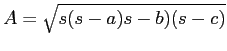where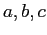are the lengths of the three sides of the triangle and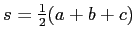. I let the sides of the triangle be represented by the three consecutive integers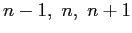. I then found, after simplification, that(1)

Upon examination of this result it was clear that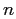had to be an even integer in order for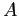to be an integer. For ifwere odd,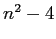would be odd and even if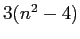were a perfect square,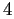would not divide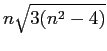. Also, by observation, it is clear thatmust be equal to a number of the form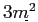. I did a quick search and found that the integers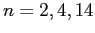all worked. Since my assumption was that there were going to be an infinite number of these triangles, I looked for a recurrence relation for which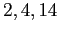were the first three terms that generated and infinite sequence of values forsatisfying (1). I would then test this sequence of to see if the values always determined a Super-Heronian triangle.

Looking at the three terms,, my guess was that these values satisfied the sequence given by the recurrence relation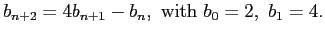(2)

I used this sequence to generate more terms, all of which seemed to work (see the table on the next page).

The sequence generated by the recurrence relation (2) can also be represented by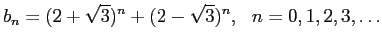(3)

This sequence can be obtained from the auxiliary equation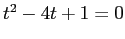which has the roots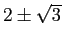. Therefore we let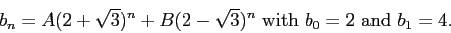Here are the first terms terms which do, in fact, generate Super-Heronian triangles. The first row generates a line which represents a degenerate triangle.

 a b c Area 1 2 3 0 3 4 5 6 13 14 15 84 51 52 53 1170 193 194 195 16296 723 724 725 226974 2701 2702 2703 3161340 10083 10084 10085 44031786 37633 37634 37635 613283664 140451 140452 140453 8541939510 524173 524174 524175 118973869476

Table 1

As one can see, the sides get large very fast. It is left to show that every term of the sequence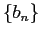yields a solution; that is, an area that is an integer. Let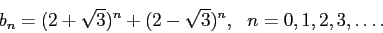Then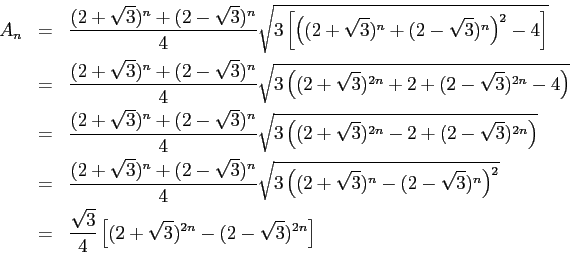Now, by using mathematical induction, this last expression can be shown to be an integer for all integers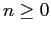. (Left as an exercise for the reader.) Hence, since the sequence is infinite, there are an infinite number of Super-Heronian triangles. It should be noted that we have not shown that our sequencegenerates all Super-Heronian triangles, but it does verify that there are an infinite number of them.

Let us examine another interesting feature of these triangles. Suppose we use the area formula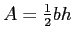. We then have the area of the triangle given by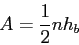whereis the length of sideand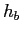is the altitude to side. Thus,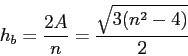which is an integer. Moreover,divides the triangle into two right triangles with sidedivided into two lengths,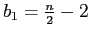and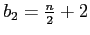. It is interesting to note that one of these right triangles has sides that are a primitive Pythagorean triple. In the table below, the asterisks mark the primitive triple in each row. Note how the primitve triples alternate.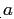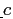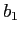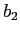1 2 3 0 - - 3 4 5* 3* 0 4* 13* 14 15 12* 5* 9 51 52 53* 45* 24 28* 193* 194 195 168* 95* 99 723 724 725* 627* 360 364* 2701* 2702 2703 2340* 1349* 1353 10083 10084 10085* 8733* 5040 5044* 37633* 37634 37635 32592* 18815* 18819 140451 140452 140453* 121635* 70224 70228* 524173* 524174 524175 453948* 262085* 262089

Table 2

One might observe that thes can be generated on their own from the recurrence relation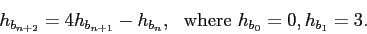Bill Richardson 2010-12-10